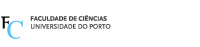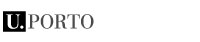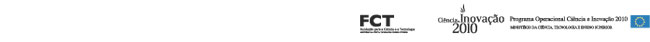# Statistical, Modeling and Computational Applications

Este seminário é organizado conjuntamente pelo GEMAC e pelas áreas de investigação do CMUP “Análise Numérica, Probabilidades e Estatística” e “Processamento de Sinal e Análise de Dados”.

# Seminar 2012

Date
Room
Speaker
Affiliation
Title
Abstract
27 Jan
1.08 DM
Isabel Pereira Departamento de Matemática da Universidade de Aveiro (UA) and CIDMA, UA Integer-valued Bilinear Time Series Models The analysis of count processes has become an important area of research in the last two decades. In this paper we consider the simple non-negative integer-valued bilinear process INBL(1,0,1,1). The parameter estimation is addressed and the problem of forecasting integer-valued time series modeled by the INBL(1,0,1,1) process is considered. Bayesian methodology is used to obtain parameter estimates, point predictions and confidence intervals for future values of the process. The parameters estimates and predictions thus obtained are compared with their classic counterparts. The proposed approaches are illustrated with a simulation study.
2 Março 2012
11h30-12h30
1.08 DM Jorge Freitas
CMUP/GEMAC
DM/FCUP
Introduction to Extreme Value Theory and its applications
In this lecture, we will present a brief introduction to Extreme Values. We will start by the classical theory, the extremal types theorem, the domains of attraction, their connection with the tail of the distribution. Then we will address the dependent case, the possible appearance of clustering and the extremal index. Finally, we will talk about the tail index, its estimation and its applications.
9 Março 2012
11h30 -12h30
1.08 DM Ana Cristina Freitas
CMUP/GEMAC
FEP
Upper bounds for ruin probabilities in the Sparre Andersen model We consider the problem of estimating the adjustment coefficient R in the Sparre Andersen model, which allows us to estimate upper bounds for the ruin probability of insurance companies.
We propose a consistent estimator for R and establish a result about its asymptotic normality. Moreover, we show that it is possible to construct confidence intervals for R based on that estimator, using the tail bootstrap procedure.
We also compare the confidence intervals computed using the normal approximations and the tail bootstrap method. We present the results of a simulation study in some particular cases.

CMUP/GEMAC
Centro de Matemática da Universidade do Porto
Edifício da Matemática da FCUP
Rua do Campo Alegre, 687
4169-007 Porto

Tel: 220 402 131
Telem: 93 319 27 63
Fax: 220 402 108
Email: gemac@fc.up.pt

Homepage:
www.fc.up.pt/cmup/gemac